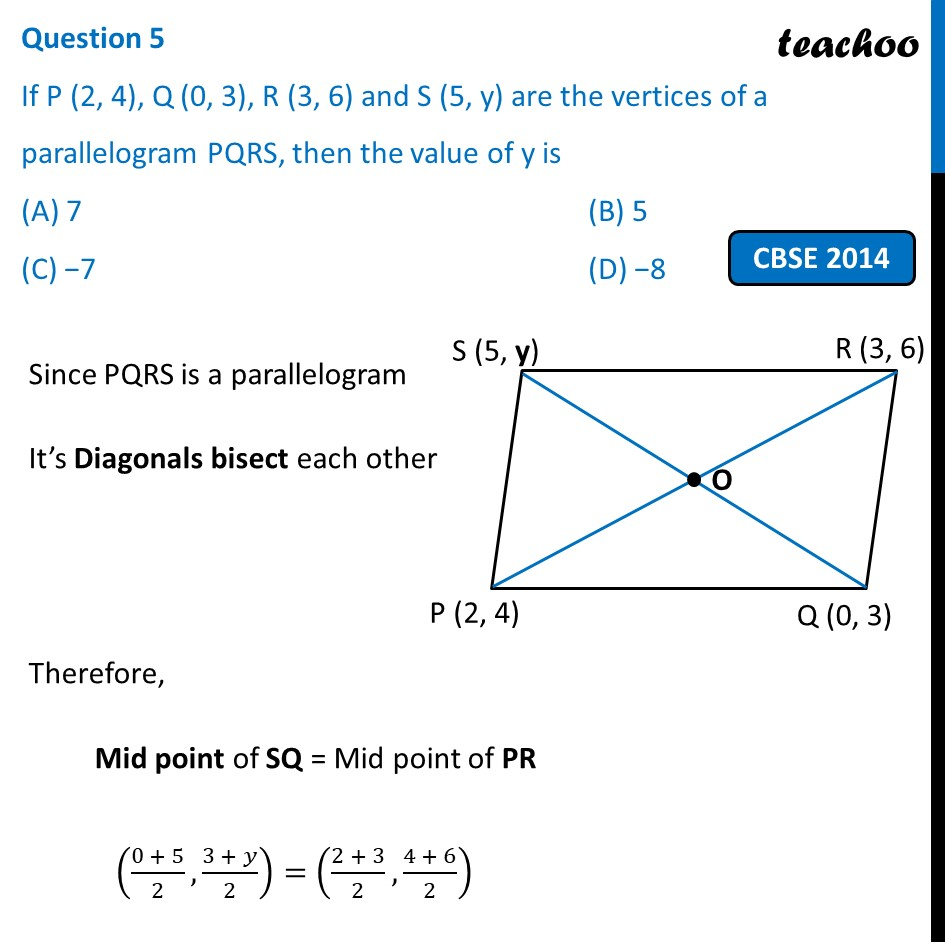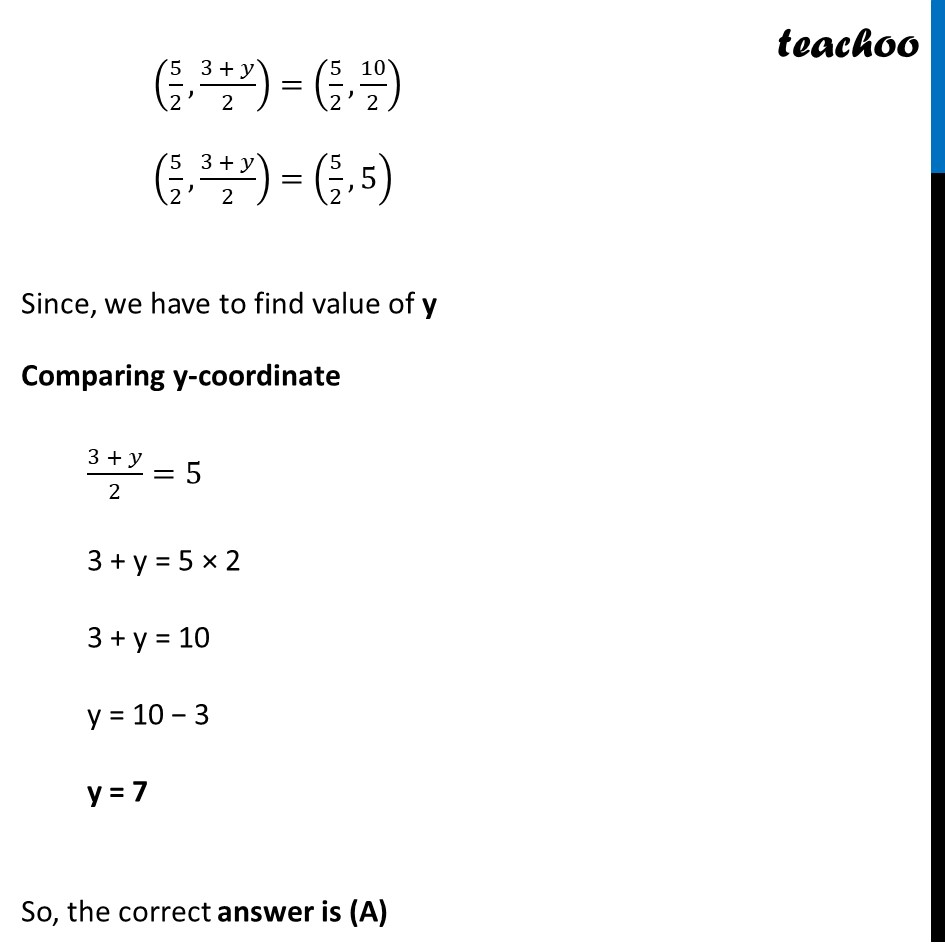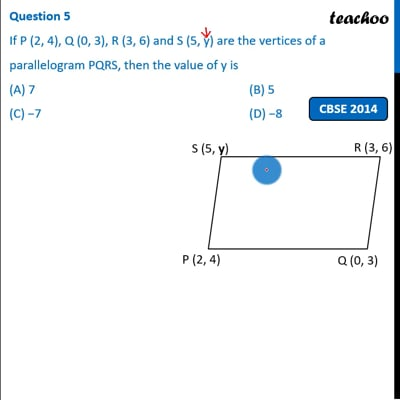Past Year MCQ

Chapter 7 Class 10 Coordinate Geometry (Term 1)
Serial order wise

## (D) −8

This question is simlar to Example 10 - Chapter 7 Class 10 Coordinate GeometryThis video is only available for Teachoo black users

### Transcript

Question 5 If P (2, 4), Q (0, 3), R (3, 6) and S (5, y) are the vertices of a parallelogram PQRS, then the value of y is (A) 7 (B) 5 (C) −7 (D) −8 Since PQRS is a parallelogram It’s Diagonals bisect each other Therefore, Mid point of SQ = Mid point of PR ((0 + 5)/2,(3 + 𝑦)/2)=((2 + 3)/2,(4 + 6)/2) (5/2,(3 + 𝑦)/2)=(5/2,10/2) (5/2,(3 + 𝑦)/2)=(5/2,5) Since, we have to find value of y Comparing y-coordinate (3 + 𝑦)/2=5 3 + y = 5 × 2 3 + y = 10 y = 10 − 3 y = 7 So, the correct answer is (A)